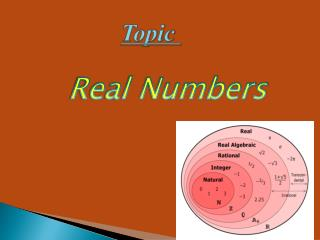DownloadDownload PresentationTopic

# Topic

Download Presentation## Topic

- - - - - - - - - - - - - - - - - - - - - - - - - - - E N D - - - - - - - - - - - - - - - - - - - - - - - - - - -
##### Presentation Transcript

1. Topic Real Numbers

2. Real Numbers • Real numbers consist of all the rational and irrational numbers. • The real number system has many subsets: • Natural Numbers • Whole Numbers • Integers

3. Natural Numbers • Natural numbers are the set of counting numbers which starts from 1. • They are denoted by N Example : {1, 2, 3,…}

4. Whole Numbers • Whole numbers are the set of numbers that include 0 plus the set of natural numbers. Example : {0, 1, 2, 3, 4, 5,…}

5. Integers An integer is a whole number (not a fractional number) that can be positive, negative, or zero. It is denoted by Z . Example : Z = {..., -3, -2, -1, 0, 1, 2, 3, ...}

6. Rational Numbers • Rational numbers are any numbers that can be expressed in the form of a/b , where a and b are integers, and b ≠ 0. • They can always be expressed by using terminating decimals or repeating decimals. • Example : 2/3, 6/7,1

7. Repeating Decimals Terminating Decimals • Repeating decimals are decimals that contain a infinite number of digits.Examples: • 0.333… , 7.689689… • Terminating decimals are decimals that contain a finite number of digits. • Examples: • 36.8 , 0.125 Non Terminating Decimals While expressing a fraction into a decimal by the division method, if the division process  continues indefinitely, and zero remainder is never obtained then such a decimal is called Non-Terminating Decimal .A non-terminating decimal is a decimal that never terminates.Example :0.076923....,  0.05882352.....

8. Euclid's Division Lemma Euclid's division lemma states that " For any two positive integers a and b, there exist integers q and r such that a= bq + r , 0≤ r< b Lemma: A lemma is a proven statement used for proving another statement Example : For a= 15,b=3 it is observed that 15=3(5)+0 where q=5 and r=0

9. Algorithm: An algorithm is a series of well defined steps which gives a procedure for solving a type of problem. Euclid division algorithm can be used to find the HCF of two numbers. It can also be used to find some common properties of numbers. To obtain the HCF of two positive integers,say c and d, with c>d , we have to follow the steps below: STEP 1: Apply Euclid division lemma, to c and d. So, we find whole numbers, q and r such that c= dq +r STEP 2: If r=0,d is the HCF of c and d. If r does not equal to 0 , apply the division lemma to d and r. STEP 3: Continue the process till the remainder is zero. The divisor at this stage will be the required HCF.

10. Question: Use Euclid algorithm to find the HCF of 455 and 42 ? Solution : Since 455>42, we apply division lemma to 455 and 42 , to get 455= 42 × 10+35 Remainder is not zero therefore we apply lemma to 42 and 35, 42=35×1+7 Again remainder is not zero therefore we apply lemma to 35 and 7 35=7 ×5+0 The remainder has become zero , and we cannot proceed any further ,therefore the HCF of 455 and 42 is the divisor at this stage , i,e 7

11. The Fundamental Theorem of Arithmetic Every composite number can be expressed as a product of primes. This representation is called prime factorisation of the number. This factorisation is unique, apart from the order in which the prime factors occur.

12. The HCF of two numbers is equal to the product of the terms containing the least powers of common prime factors of the two numbers. Highest Common Factor(HCF): The LCM of two numbers is equal to the product of the terms containing the greatest powers of all prime factors of the two numbers. Lowest Common Multiple(LCM):

13. Relationship between HCF and LCM Example : if a=3 and b=6 HCF(3,6)× LCM(3,6) = 3× 6 3 × 6 = 18 18 = 18 Hence verified……..

14. Question : Given that HCF(306,657)=9, find LCM(306,657) Solution : We know that the product of the HCF and the LCM of two numbers is equal to the product of the given numbers . × × × × Therefore HCF(306,657) LCM(306,657) = 306 657 9 LCM(306,657) = 306 657 LCM(306,657) = 306 657/9 LCM =22338 ×

15. Irrational Numbers • Irrational numbers are any numbers that cannot be expressed as a/b. • They are expressed as non-terminating, non-repeating decimals; decimals that go on forever without repeating a pattern. • Examples of irrational numbers: • 0.34334333433334… • 45.86745893… • π

16. Things to Remember . Let p be a prime number. If p divides a 2 then p also divides a, where a is a positive integer. . When prime factorisation of q is of the form 2m5n.Then x has a decimal expansion which terminates and when q is not of the form 2m5n, then x has a decimal expansion which is non-terminating repeating or recurring.

17. . Is 17/8 has a terminating decimal expansion? Sol : We have 17/8 =17/23 So , the denominator 8 of 17/8 is of the form 2m×5n therefore it has a terminating decimal expansion. .Is 29/343 has a terminating decimal expansion? Sol : We have 29/343 =29/35 Clearly 343 is not of the form 2m×5n therefore it has a non terminating decimal expansion.